# Common fractions and decimals#### All in One Place

Everything you need for better grades in university, high school and elementary.#### Learn with Ease

Made in Canada with help for all provincial curriculums, so you can study in confidence.#### Instant and Unlimited Help

0/5
##### Intros
###### Lessons
1. Introduction to Common Fractions and Decimals:
2. How are decimals related to fractions?
3. How to convert decimal tenths into decimal fractions ($\large \frac{1}{10}$)
4. How to convert decimal hundredths into decimal fractions ($\large \frac{1}{100}$)
5. How to convert between decimals and common fraction halves ($\large \frac{1}{2}$)
6. How to convert between decimals and common fraction quarters ($\large \frac{1}{4}$)
0/13
##### Examples
###### Lessons
1. Writing common fractions as decimals
Write the following fractions as decimals
1. Unit fractions:
2. Decimal fractions:
3. Common fractions related to $\large \frac{1}{2}$ or $\large \frac{1}{4}$:
2. Fraction and figure equivalents for decimals
Complete the table for the equivalent decimal, fraction, and shaded in figure:
1.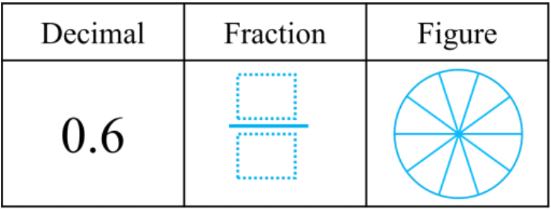2.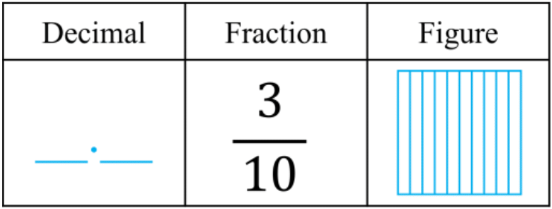3.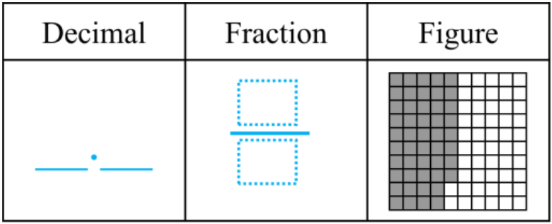4.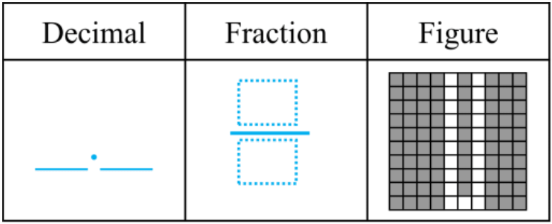3. Fraction and figure equivalents for decimals
Complete the table for the equivalent decimal, fraction, and shaded in figure:
1. 0.28 = $\large \frac{}{100}$ = $\large \frac{20}{100}$ + $\large \frac{}{100}$ = $\large \frac{}{10}$ + $\large \frac{}{100}$
2. 0.51 = $\large \frac{}{100}$ = $\large \frac{50}{100}$ + $\large \frac{}{100}$ = $\large \frac{}{10}$ + $\large \frac{}{100}$
3. 0.73 = $\large \frac{?}{?}$ = $\large \frac{}{100}$ + $\large \frac{}{100}$ = $\large \frac{}{10}$ + $\large \frac{}{100}$
4. 0.49 = $\large \frac{?}{?}$ = $\large \frac{?}{?}$ + $\large \frac{?}{?}$ = $\large \frac{?}{?}$ + $\large \frac{?}{?}$
4. Common fractions and decimal number lines
Using common fractions and decimals:

A:$\large \frac{25}{100} \qquad \;$ B:$\large \frac{7}{10} \qquad \;$ C:$\large \frac{90}{100} \qquad \;$ D:$\large \frac{5}{10} \qquad \;$ A:$\large \frac{75}{100} \qquad$
1. Draw each fraction as a point on the number line. Label each point with its corresponding letter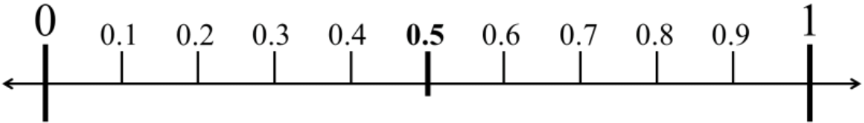2. Which fractions can be rewritten in lowest forms as common fraction halves and quarters?
0%
##### Practice
###### Topic Notes

In this lesson, we will learn:

• How to convert between decimals and decimal fractions (tenths and hundredths)
• How to convert between decimals and unit fractions
• How to convert between decimals and fraction halves and quarters

Notes:

• Decimals can be converted into fractions and vice versa
• Recall that place values are related to their neighbors by a factor of 10

•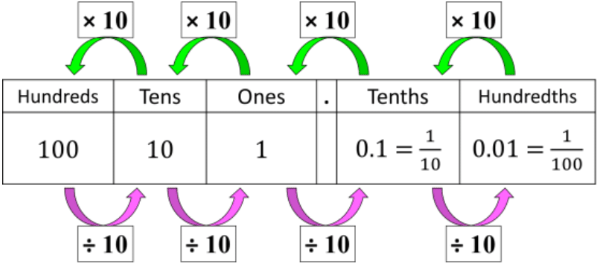• Decimals can be easily converted into decimal fractions
• Decimal fractions have denominators that are powers of 10
• Decimal tenths are fractions out of 10
• Ex. 0.3 = $\large \frac{3}{10}$; 3 tenths = 3 out of 10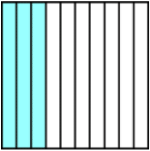• Decimal hundredths are fractions out of 100
• Ex. 0.22 = $\large \frac{22}{100}$; 22 hundredths = 22 out of 100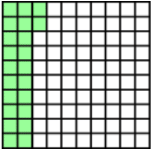• Decimal hundredths can also be expressed as a sum of fractions, because of equivalent decimals (i.e. 0.2 = 0.20)
• 0.22 = $\large \frac{22}{100}$ = $\large \frac{20}{100}$ + $\large \frac{2}{100}$ = $\large \frac{2}{10}$ + $\large \frac{2}{100}$

• Two type of common fractions have a denominator of 2 or 4

 Type Fraction Decimal Value "half" $\frac{1}{2}$ = $\frac{50}{100}$ = $\frac{5}{10}$ 0.5 "quarter" $\frac{1}{4}$ = $\frac{25}{100}$ 0.25